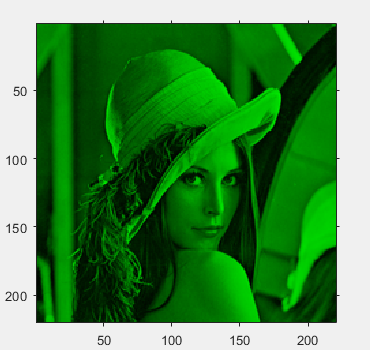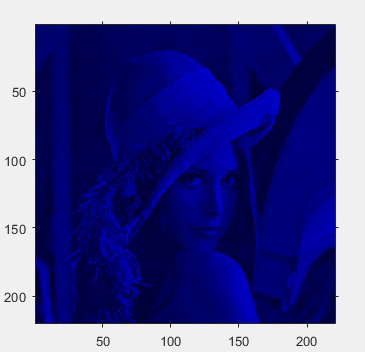# Display the red, green and blue color planes of a color image in MATLAB

Prerequisite – RGB image representation
A colored image can be represented as a 3 order matrix. The first order is for the rows, the second order is for the columns and the third order is for specifying the color of the corresponding pixel. Here we use the RGB color format, so the third order will take 3 values of Red, Green ans Blue respectively. The values of the rows and columns depends on the size of the image.

Approach:

• Store the number of rows and columns of the image in variables, r and c.
• Create 3 zero matrices R, G and B (one for each of the 3 colors) of size rXc.
• Store the corresponding color plane of the image in the corresponding zero matrix.
```1: Red
2: Green
3: Blue ```
• Display the images by using imshow(), but typecast them into uint8 first.

Implementation:

 `% MATLAB code to display the red, green and blue ` `% color planes of a color image ` ` `  `% read the image ` `I = imread(``'lenna.png'``); ` ` `  `% rows and columns in the image ` `r = size(I, 1); ` `c = size(I, 2); ` ` `  `% creating zero matrices ` `R = zeros(r, c, 3); ` `G = zeros(r, c, 3); ` `B = zeros(r, c, 3); ` ` `  `% storing the corresponding color plane ` ` `  `% red plane ` `R(:, :, 1) = I(:, :, 1); ` ` `  `% green plane ` `G(:, :, 2) = I(:, :, 2); ` ` `  `% blue plane ` `B(:, :, 3) = I(:, :, 3); ` ` `  `% displaying the images ` `figure, imshow(uint8(R)); ` `figure, imshow(uint8(G)); ` `figure, imshow(uint8(B)); `

Input :Output :My Personal Notes arrow_drop_upCheck out this Author's contributed articles.

If you like GeeksforGeeks and would like to contribute, you can also write an article using contribute.geeksforgeeks.org or mail your article to contribute@geeksforgeeks.org. See your article appearing on the GeeksforGeeks main page and help other Geeks.

Please Improve this article if you find anything incorrect by clicking on the "Improve Article" button below.

Article Tags :

Be the First to upvote.

Please write to us at contribute@geeksforgeeks.org to report any issue with the above content.